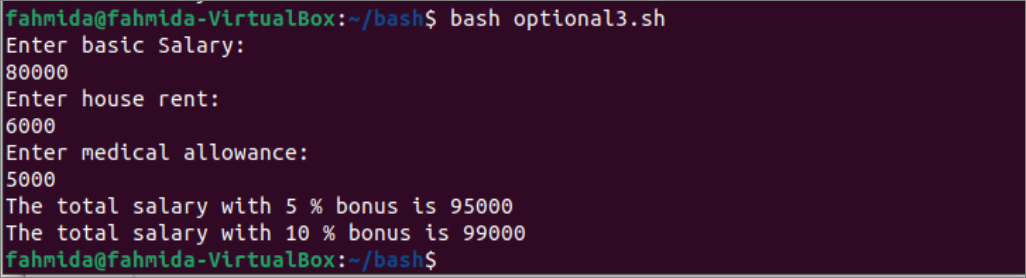Gice

Technology and General BlogA function is an essential part of any programming language that contains a block of code. The same code can be executed multiple times by calling a function, and the requirement to write the same code repeatedly can be avoided by using the function. A function can be defined without argument and with argument. The argument of the function can be mandatory and optional. The default value can be set for the argument of the bash function. The ways to declare the optional argument and the use of default values in the bash function have shown in this tutorial.

## Example-1:Calculate sum using optional Arguments

The way to define optional arguments with default values is shown in this example. Create a bash file with the following script to calculate the sum of two numbers. In the script, the function named sum() contains two optional arguments with default values. If no argument is given when calling the function, the sum of the default values will be calculated. If one argument is given when calling the function, then the sum of the argument value and the second default value will be calculated. If two arguments are given at the time of calling the function, then the sum of the argument values will be calculated. Next, the function has called without any arguments, with one argument, and with two arguments.

#!/bin/bash
#Declare function with mandatory and optional argument
functionsum()

#Set the values
num1=\$1:-10
num2=\$2:-20

#Return true if no argument is given
if [ \$# -lt1 ]; then
echo “The optional argument values are: \$num1, \$num2.”
#Return true if one argument is given
elif [ \$# -lt2 ]; then
echo
echo “The optional argument value is: \$num2.”
else
echo
echo “There is no optional argument.”
num1=\$1
num2=\$2
fi
#Calculate the sum of two numbers
sum=\$((num1+num2))
echo “The sum of \$num1 and \$num2 is \$sum

#Call function without any argument
sum
#Call function with one argument
sum 40
#Call function with two arguments
sum 70 30

Output:

The following output will appear after executing the above script. When the function was called without any argument, the sum of two default values was printed that 30(10+20). When the function was called with one argument, the sum of the argument value(40) and the second default value(20) is printed that is 60. When the function was called with two arguments, the sum of two argument values has printed 100(70+30).

## Example-2: Authenticate the user using

default values

#!/bin/bash
#Declare function for authentication
functionAuthenticate()

#Set the values

#Return true if no argument is given
echo “You have logged in as Administrator.”
#Return true if one argument is given
echo “You have logged in as Guest.”
else
fi

#Call function without any argument
Authenticate
Authenticate fahmida 1234

Output:

The following output will appear after executing the above script.## Example-3: Calculate the bonus based on the default value

Create a bash file with the following script to calculate the bonus based on the default value if no argument value is given for the function. Three input values will be taken from the user after executing the script. These are basic salary, house rent, and medical allowance. The function named calculate_salary() will calculate the bonus amount based on the argument value of the function or the default value. The total salary amount will be counted by adding the values of basic, rent, medical, and bonusAmount. The calculate_salary() function has called without any argument or with an argument.

#!/bin/bash

#Take basic, house rent and medical allowance of an employee
echo “Enter basic Salary:”
echo “Enter house rent:”
echo “Enter medical allowance:”

#Declare function to calculate salary with bonus
functioncalculate_salary()

#Set the value
bonus=\$1:-5
#Calculate bonus
bonusAmount=\$((basic*bonus/100))
#Calculate total salary
total=\$((basic+rent+medical+bonusAmount))
#Print total salary with the bonus amount
echo “The total salary with \$bonus % bonus is \$total

#Call function without the percentage of bonus
calculate_salary
#Call function with the percentage of bonus
calculate_salary 10

Output:

The following output will appear after executing the script. When the function has been called without any argument, the default value 5 has been used to calculate the bonus amount based on the basic amount. When the function has called with the argument value 10, the bonus amount has been calculated based on this value. Next, the total salary based on 5% bonus and 10% bonus has printed.## Conclusion:

The use of optional arguments with default values in the function has been described in this tutorial using three different examples. The default value can be numeric or string. The purpose of using an optional argument with the default values in the function will be cleared for the bash user after reading this tutorial.